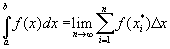# Riemann Sums Applet

For continuous functions, the definite Riemann Integral can be defined asIf we do not evaluate the limit, and instead only use a finite n, the Riemann Sum will only be an approximation of the exact value of the integral. While in the limit, it does not matter how the sample points, x*, are chosen, for a finite n, the numerical approximation may have different properties. The sample points can be chosen as: left endpoint of the interval, the right endpoint, or the midpoint. Additionally, another numerical method, the trapezoid rule is used. Technically, the trapezoid rule is not a Riemann sum, but it is a method of numerical integration used to approximate definite integrals